## Techniques of Indefinite Integration

Integration by substitution. This section opens with integration by substitution, the most widely used integration technique, illustrated by several examples. The idea is simple: Simplify an integral by letting a single symbol (say the letter u) stand for some complicated expression in the integrand. If the differential of u is left over in the integrand, the process will be a success.

Example 1: DetermineLet u = x 2 + 1 (this is the substitution); then du = 2 x dx, and the given integral is transformed intowhich transforms back to ⅓( x 2 + 1) 3/2; + c.

Example 2: Integrate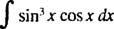Let u = sin x; then du = cos x dx, and the given integral becomes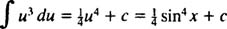Example 3: EvaluteFirst, rewrite tan x as sin x/cos x; then let u = cos x, du = − sin x dx:Example 4: Evaluate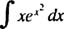Let u = x 2; then du = 2 x dx, and the integral is transformed intoExample 5: DetermineLet u = sec x; then du = sec x dx, and the integral is transformed into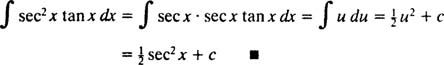Integration by parts. The product rule for differentiation says d( uv) = u dv + v du. Integrating both sides of this equation gives uv = ∫ u dv + ∫ v du, or equivalentlyThis is the formula for integration by parts. It is used to evaluate integrals whose integrand is the product of one function ( u) and the differential of another ( dv). Several examples follow.

Example 6: Integrate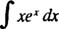Compare this problem with Example 4. A simple substitution made that integral trivial; unfortunately, such a simple substitution would be useless here. This is a prime candidate for integration by parts, since the integrand is the product of a function ( x) and the differential ( e x dx) of another, and when the formula for integration by parts is used, the integral that is left is easier to evaluate (or, in general, at least not more difficult to integrate) than the original.

Let u = x and dv = e x dx; then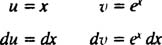and the formula for integration by parts yields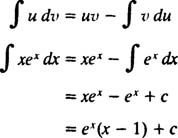Example 7: IntegrateLet u = x and dv = cos x dx; then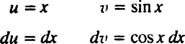The formula for integration by parts gives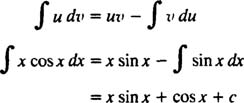Example 8: Evaluate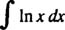Let u = In x and dv = dx; thenand the formula for integration by parts yieldsBack to Top
A18ACD436D5A3997E3DA2573E3FD792A